# Forum: Wishes and new features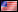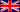Features you would like to see in VirtualDJ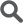Topic: More accurate VU meters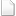The VU Meters should be numbered to know where the zero dB is and see the headroom and clip in different colors, for example green, yellow and red.

Posted Mon 17 Aug 20 @ 6:09 pm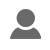VDJ Monty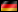PRO Infinity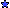Member since 2020+1

and:
The meter should be configurable. At many controllers its usual: 1 LED = 3dB

Posted Mon 17 Aug 20 @ 7:41 pmymed85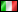PRO InfinityMember since 2020+1

Posted Sat 29 Aug 20 @ 10:19 am+1

Posted Sat 29 Aug 20 @ 12:43 pmVDJ MontyPRO InfinityMember since 2020Quote :
The VU Meters should be numbered to know where the zero dB is and see the headroom and clip in different colors, for example green, yellow and red.

For me, I've added some markes into the skin. And ive changed the colours at the image file of the skin.
But, the scale seems to be hard coded, i cannot change it. It seems to be between 0db and -24dB. -72dB would be fine.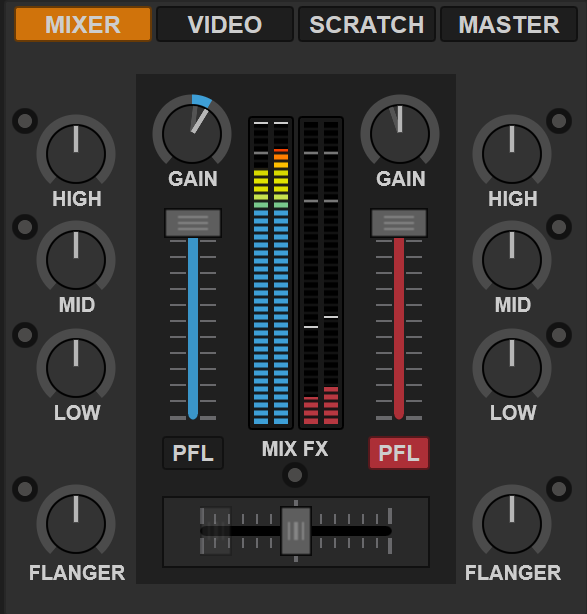Posted Fri 16 Oct 20 @ 12:47 pmthere is a possibility to change colors :
vu_meters are actually hardcoded in bitmap linear visuals
they can be vectorized using leds and vumeter visual
by the way the led will be set fully instead of partially

<define class="vumeter" type="vumeter" orientation="vertical" direction="up" width="11" height="201" granularity="41">
<led x="+0" y="+5*40+2" width="11" height="5"><off color="vumeterdarker" border="black" border_size="1" radius="1"/><on color="deckcolor" border="black" border_size="1" radius="1"/></led>
<led x="+0" y="+5*39+2" width="11" height="5"><off color="vumeterdarker" border="black" border_size="1" radius="1"/><on color="deckcolor" border="black" border_size="1" radius="1"/></led>
...
<led x="+0" y="+5*01+2" width="11" height="5"><off color="vumeterlighter" border="black" border_size="1" radius="1"/><on color="vumeterhigh" border="black" border_size="1" radius="1"/></led>
<led x="+0" y="+5*00+2" width="11" height="5"><off color="vumeterlighter" border="black" border_size="1" radius="1"/><on color="vumeterhigh" border="black" border_size="1" radius="1"/></led>
</define>

...
<visual class="vumeter" deck="left" source="get_vu_meter_left" x="+0" y="+0"/>
...

every led on and off can have its owv color

Posted Fri 16 Oct 20 @ 2:12 pmGreat Job. That's what I'm talking about.

Posted Fri 16 Oct 20 @ 8:58 pmNicotux wrote :
there is a possibility to change colors :
vu_meters are actually hardcoded in bitmap linear visuals
they can be vectorized using leds and vumeter visual
by the way the led will be set fully instead of partially

<define class="vumeter" type="vumeter" orientation="vertical" direction="up" width="11" height="201" granularity="41">
<led x="+0" y="+5*40+2" width="11" height="5"><off color="vumeterdarker" border="black" border_size="1" radius="1"/><on color="deckcolor" border="black" border_size="1" radius="1"/></led>
<led x="+0" y="+5*39+2" width="11" height="5"><off color="vumeterdarker" border="black" border_size="1" radius="1"/><on color="deckcolor" border="black" border_size="1" radius="1"/></led>
...
<led x="+0" y="+5*01+2" width="11" height="5"><off color="vumeterlighter" border="black" border_size="1" radius="1"/><on color="vumeterhigh" border="black" border_size="1" radius="1"/></led>
<led x="+0" y="+5*00+2" width="11" height="5"><off color="vumeterlighter" border="black" border_size="1" radius="1"/><on color="vumeterhigh" border="black" border_size="1" radius="1"/></led>
</define>

...
<visual class="vumeter" deck="left" source="get_vu_meter_left" x="+0" y="+0"/>
...

every led on and off can have its owv color

TKS, I'll try...

Posted Fri 16 Oct 20 @ 9:01 pmHeltinoPRO InfinityMember since 2020+1
On my DDJ-RX are the VU meters, auto gain is turned on, pointless as just 1 LED is doing something.
Would love to have them working correct to a) validate the aktual gain and b) having the optical help on which channel is something running on the controller itself

Posted Mon 19 Oct 20 @ 2:51 pm